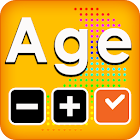Age & Time Calculator

All Android applications categories

All Android games categories# Age & Time Calculator

by: 309 7.6

7.6 Users
rating

## Screenshots

Description

Age calculator and time calculator.

1. Age Calculator
Is it difficult to calculate this when you write your government office documents and resume?

You can calculate the number of days you have lived.

Calculate the number of baby months.
Try using the age calculator.

2. Time calculator
What year is a year?
How many minutes a year?

How many years is ten thousand hours?

Use the time calculator here.
Here, use the time converter, time calculator.

from 309 reviews

"Great"

7.6